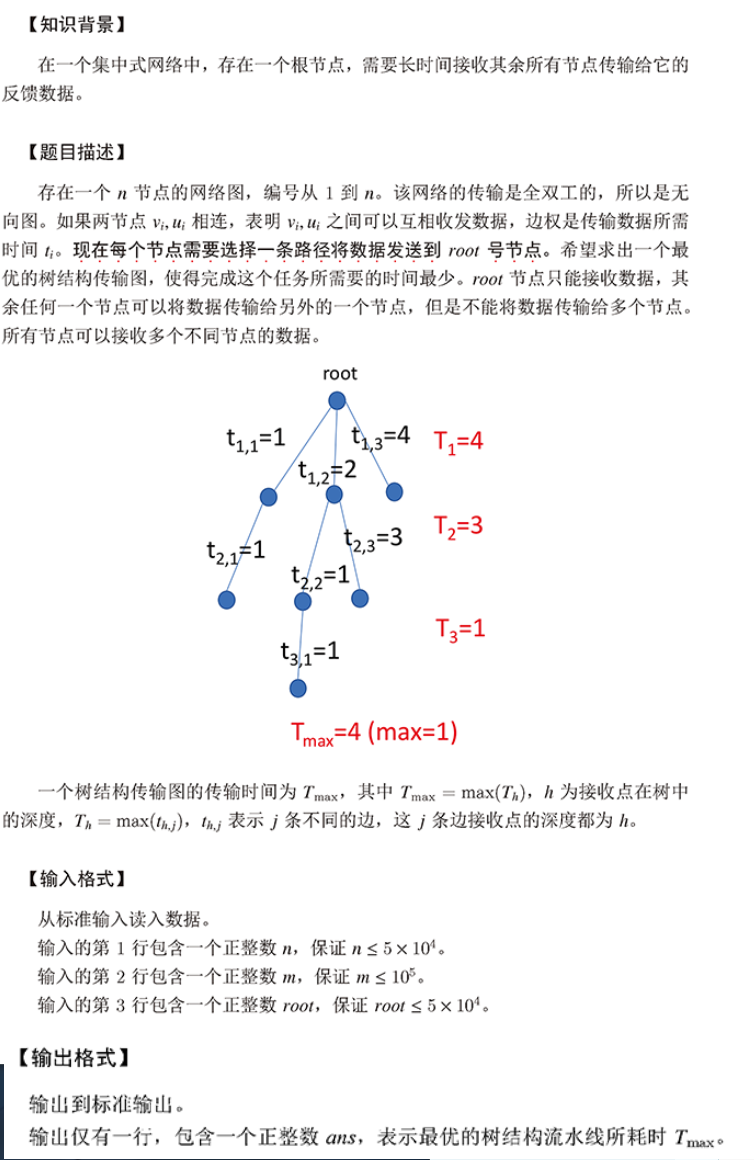# 程序设计思维与实践 Week6 作业 D 数据中心

### 题目描述：### 思路：

#include<iostream>
#include<algorithm>
using namespace std;
const int maxx=100010;
int n,m,root,tot,ans=-0x7f,father[maxx];
struct node
{
int u;
int v;
int w;
bool operator <(const node&o)const
{
return w<o.w;
}
}e[maxx];
int find(int x)
{
if(x!=father[x])
father[x]=find(father[x]);
return father[x];
}
void unionn(int a,int b)
{
int fa=find(a);
int fb=find(b);
father[fa]=fb;
}
int main()
{
cin>>n>>m>>root;
for(int i=1;i<=m;i++)
cin>>e[i].u>>e[i].v>>e[i].w;
for(int i=1;i<=n;i++)
father[i]=i;
sort(e+1,e+m+1);
for(int i=1;i<=m;i++)
{
if(find(e[i].u)!=find(e[i].v))
{
unionn(e[i].u,e[i].v);
ans=max(ans,e[i].w);
tot++;
}
if(tot==n-1)
break;
}
cout<<ans;
return 0;
}

©️2019 CSDN 皮肤主题: 数字20 设计师: CSDN官方博客